## 15.2 Energy in Simple Harmonic Motion

### Learning Objectives

By the end of this section, you will be able to:

• Describe the energy conservation of the system of a mass and a spring
• Explain the concepts of stable and unstable equilibrium points

To produce a deformation in an object, we must do work. That is, whether you pluck a guitar string or compress a car’s shock absorber, a force must be exerted through a distance. If the only result is deformation, and no work goes into thermal, sound, or kinetic energy, then all the work is initially stored in the deformed object as some form of potential energy.

Consider the example of a block attached to a spring on a frictionless table, oscillating in SHM. The force of the spring is a conservative force (which you studied in the chapter on potential energy and conservation of energy), and we can define a potential energy for it. This potential energy is the energy stored in the spring when the spring is extended or compressed. In this case, the block oscillates in one dimension with the force of the spring acting parallel to the motion:

$W=\underset{{x}_{i}}{\overset{{x}_{f}}{\int }}{F}_{x}dx=\underset{{x}_{i}}{\overset{{x}_{f}}{\int }}\text{−}kxdx={[-\frac{1}{2}k{x}^{2}]}_{{x}_{i}}^{{x}_{f}}=\text{−}[\frac{1}{2}k{x}_{f}^{2}-\frac{1}{2}k{x}_{i}^{2}]=\text{−}[{U}_{f}-{U}_{i}]=\text{−}\text{Δ}U.$

When considering the energy stored in a spring, the equilibrium position, marked as ${x}_{i}=0.00\,\text{m,}$ is the position at which the energy stored in the spring is equal to zero. When the spring is stretched or compressed a distance x, the potential energy stored in the spring is

$U=\frac{1}{2}k{x}^{2}.$

### Energy and the Simple Harmonic Oscillator

To study the energy of a simple harmonic oscillator, we need to consider all the forms of energy. Consider the example of a block attached to a spring, placed on a frictionless surface, oscillating in SHM. The potential energy stored in the deformation of the spring is

$U=\frac{1}{2}k{x}^{2}.$

In a simple harmonic oscillator, the energy oscillates between kinetic energy of the mass $K=\frac{1}{2}m{v}^{2}$ and potential energy $U=\frac{1}{2}k{x}^{2}$ stored in the spring. In the SHM of the mass and spring system, there are no dissipative forces, so the total energy is the sum of the potential energy and kinetic energy. In this section, we consider the conservation of energy of the system. The concepts examined are valid for all simple harmonic oscillators, including those where the gravitational force plays a role.

Consider (Figure), which shows an oscillating block attached to a spring. In the case of undamped SHM, the energy oscillates back and forth between kinetic and potential, going completely from one form of energy to the other as the system oscillates. So for the simple example of an object on a frictionless surface attached to a spring, the motion starts with all of the energy stored in the spring as elastic potential energy. As the object starts to move, the elastic potential energy is converted into kinetic energy, becoming entirely kinetic energy at the equilibrium position. The energy is then converted back into elastic potential energy by the spring as it is stretched or compressed. The velocity becomes zero when the kinetic energy is completely converted, and this cycle then repeats. Understanding the conservation of energy in these cycles will provide extra insight here and in later applications of SHM, such as alternating circuits.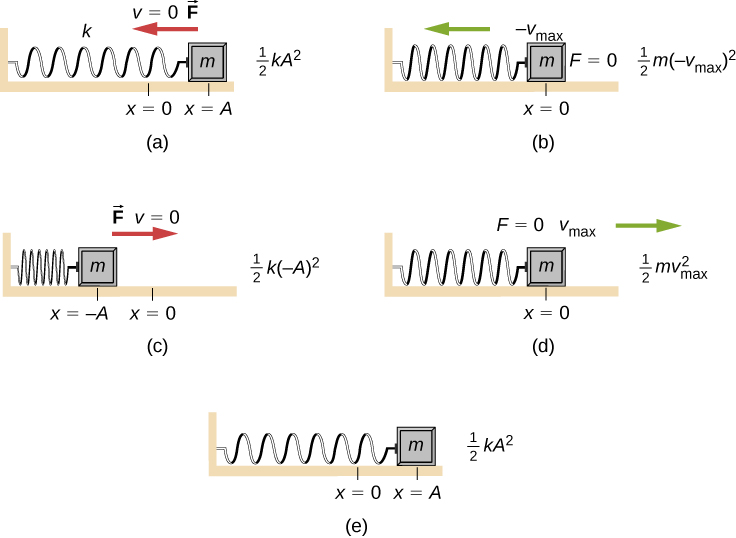Figure 15.10 The transformation of energy in SHM for an object attached to a spring on a frictionless surface. (a) When the mass is at the position $x=+A$, all the energy is stored as potential energy in the spring $U=\frac{1}{2}k{A}^{2}$. The kinetic energy is equal to zero because the velocity of the mass is zero. (b) As the mass moves toward $x=\text{−}A$, the mass crosses the position $x=0$. At this point, the spring is neither extended nor compressed, so the potential energy stored in the spring is zero. At $x=0$, the total energy is all kinetic energy where $K=\frac{1}{2}m{(\text{−}{v}_{\text{max}})}^{2}$. (c) The mass continues to move until it reaches $x=\text{−}A$ where the mass stops and starts moving toward $x=+A$. At the position $x=\text{−}A$, the total energy is stored as potential energy in the compressed $U=\frac{1}{2}k{(\text{−}A)}^{2}$ and the kinetic energy is zero. (d) As the mass passes through the position $x=0$, the kinetic energy is $K=\frac{1}{2}m{v}_{\text{max}}^{2}$ and the potential energy stored in the spring is zero. (e) The mass returns to the position $x=+A$, where $K=0$ and $U=\frac{1}{2}k{A}^{2}$.

Consider (Figure), which shows the energy at specific points on the periodic motion. While staying constant, the energy oscillates between the kinetic energy of the block and the potential energy stored in the spring:

${E}_{\text{Total}}=U+K=\frac{1}{2}k{x}^{2}+\frac{1}{2}m{v}^{2}.$

The motion of the block on a spring in SHM is defined by the position $x(t)=A\text{cos}(\omega t+\varphi )$ with a velocity of $v(t)=\text{−}A\omega \text{sin}(\omega t+\varphi )$. Using these equations, the trigonometric identity ${\text{cos}}^{2}\theta +{\text{sin}}^{2}\theta =1$ and $\omega =\sqrt{\frac{k}{m}}$, we can find the total energy of the system:

$\begin{array}{cc}\hfill {E}_{\text{Total}}& =\frac{1}{2}k{A}^{2}{\text{cos}}^{2}(\omega t+\varphi )+\frac{1}{2}m{A}^{2}{\omega }^{2}{\text{sin}}^{2}(\omega t+\varphi )\hfill \\ & =\frac{1}{2}k{A}^{2}{\text{cos}}^{2}(\omega t+\varphi )+\frac{1}{2}m{A}^{2}(\frac{k}{m}){\text{sin}}^{2}(\omega t+\varphi )\hfill \\ & =\frac{1}{2}k{A}^{2}{\text{cos}}^{2}(\omega t+\varphi )+\frac{1}{2}k{A}^{2}{\text{sin}}^{2}(\omega t+\varphi )\hfill \\ & =\frac{1}{2}k{A}^{2}({\text{cos}}^{2}(\omega t+\varphi )+{\text{sin}}^{2}(\omega t+\varphi ))\hfill \\ & =\frac{1}{2}k{A}^{2}.\hfill \end{array}$

The total energy of the system of a block and a spring is equal to the sum of the potential energy stored in the spring plus the kinetic energy of the block and is proportional to the square of the amplitude ${E}_{\text{Total}}=(1\text{/}2)k{A}^{2}.$ The total energy of the system is constant.

A closer look at the energy of the system shows that the kinetic energy oscillates like a sine-squared function, while the potential energy oscillates like a cosine-squared function. However, the total energy for the system is constant and is proportional to the amplitude squared. (Figure) shows a plot of the potential, kinetic, and total energies of the block and spring system as a function of time. Also plotted are the position and velocity as a function of time. Before time $t=0.0\,\text{s,}$ the block is attached to the spring and placed at the equilibrium position. Work is done on the block by applying an external force, pulling it out to a position of $x=+A$. The system now has potential energy stored in the spring. At time $t=0.00\,\text{s,}$ the position of the block is equal to the amplitude, the potential energy stored in the spring is equal to $U=\frac{1}{2}k{A}^{2}$, and the force on the block is maximum and points in the negative x-direction $({F}_{S}=\text{−}kA)$. The velocity and kinetic energy of the block are zero at time $t=0.00\,\text{s}\text{.}$ At time $t=0.00\,\text{s,}$ the block is released from rest.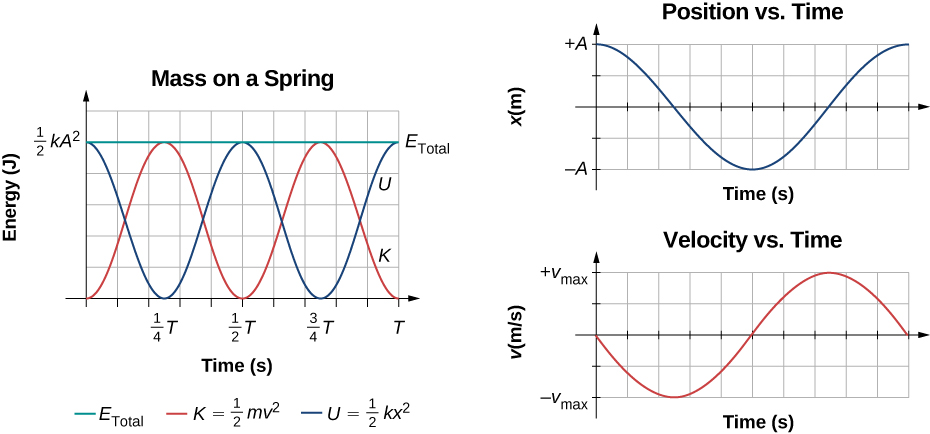Figure 15.12 Graph of the kinetic energy, potential energy, and total energy of a block oscillating on a spring in SHM. Also shown are the graphs of position versus time and velocity versus time. The total energy remains constant, but the energy oscillates between kinetic energy and potential energy. When the kinetic energy is maximum, the potential energy is zero. This occurs when the velocity is maximum and the mass is at the equilibrium position. The potential energy is maximum when the speed is zero. The total energy is the sum of the kinetic energy plus the potential energy and it is constant.

### Oscillations About an Equilibrium Position

We have just considered the energy of SHM as a function of time. Another interesting view of the simple harmonic oscillator is to consider the energy as a function of position. (Figure) shows a graph of the energy versus position of a system undergoing SHM.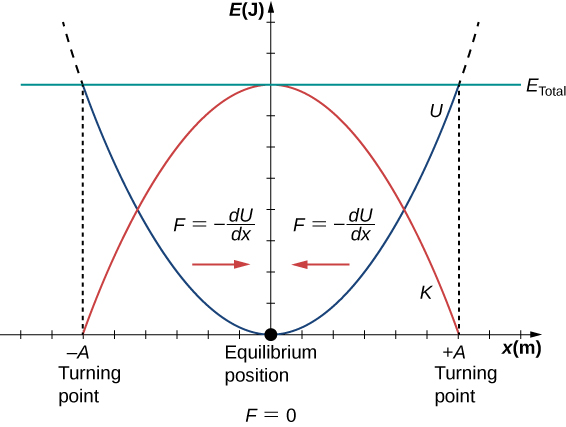Figure 15.13 A graph of the kinetic energy (red), potential energy (blue), and total energy (green) of a simple harmonic oscillator. The force is equal to $F=-\frac{dU}{dx}$. The equilibrium position is shown as a black dot and is the point where the force is equal to zero. The force is positive when $x<0$, negative when $x>0$, and equal to zero when $x=0$.

The potential energy curve in (Figure) resembles a bowl. When a marble is placed in a bowl, it settles to the equilibrium position at the lowest point of the bowl $(x=0)$. This happens because a restoring force points toward the equilibrium point. This equilibrium point is sometimes referred to as a fixed point. When the marble is disturbed to a different position $(x=+A)$, the marble oscillates around the equilibrium position. Looking back at the graph of potential energy, the force can be found by looking at the slope of the potential energy graph $(F=-\frac{dU}{dx})$. Since the force on either side of the fixed point points back toward the equilibrium point, the equilibrium point is called a stable equilibrium point. The points $x=A$ and $x=\text{−}A$ are called the turning points. (See Potential Energy and Conservation of Energy.)

Stability is an important concept. If an equilibrium point is stable, a slight disturbance of an object that is initially at the stable equilibrium point will cause the object to oscillate around that point. The stable equilibrium point occurs because the force on either side is directed toward it. For an unstable equilibrium point, if the object is disturbed slightly, it does not return to the equilibrium point.

Consider the marble in the bowl example. If the bowl is right-side up, the marble, if disturbed slightly, will oscillate around the stable equilibrium point. If the bowl is turned upside down, the marble can be balanced on the top, at the equilibrium point where the net force is zero. However, if the marble is disturbed slightly, it will not return to the equilibrium point, but will instead roll off the bowl. The reason is that the force on either side of the equilibrium point is directed away from that point. This point is an unstable equilibrium point.

(Figure) shows three conditions. The first is a stable equilibrium point (a), the second is an unstable equilibrium point (b), and the last is also an unstable equilibrium point (c), because the force on only one side points toward the equilibrium point.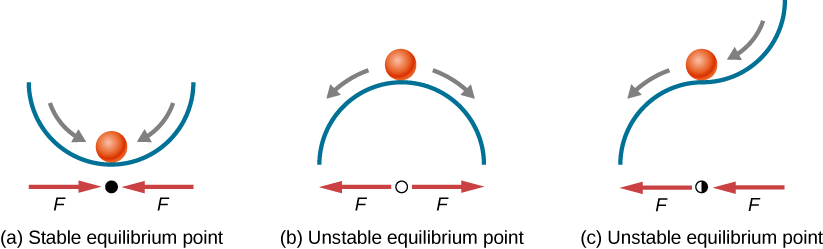Figure 15.14 Examples of equilibrium points. (a) Stable equilibrium point; (b) unstable equilibrium point; (c) unstable equilibrium point (sometimes referred to as a half-stable equilibrium point).

The process of determining whether an equilibrium point is stable or unstable can be formalized. Consider the potential energy curves shown in (Figure). The force can be found by analyzing the slope of the graph. The force is $F=-\frac{dU}{dx}.$ In (a), the fixed point is at $x=0.00\,\text{m}\text{.}$ When $x<0.00\,\text{m,}$ the force is positive. When $x>0.00\,\text{m,}$ the force is negative. This is a stable point. In (b), the fixed point is at $x=0.00\,\text{m}\text{.}$ When $x<0.00\,\text{m,}$ the force is negative. When $x>0.00\,\text{m,}$ the force is also negative. This is an unstable point.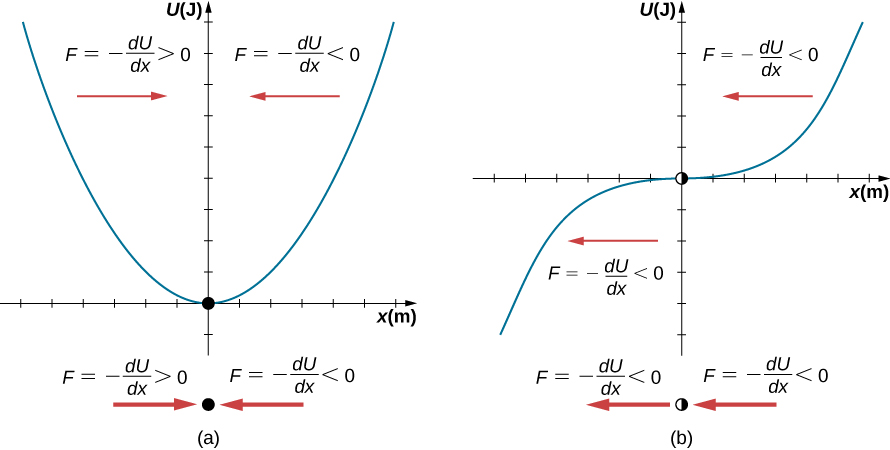Figure 15.15 Two examples of a potential energy function. The force at a position is equal to the negative of the slope of the graph at that position. (a) A potential energy function with a stable equilibrium point. (b) A potential energy function with an unstable equilibrium point. This point is sometimes called half-stable because the force on one side points toward the fixed point.

A practical application of the concept of stable equilibrium points is the force between two neutral atoms in a molecule. If two molecules are in close proximity, separated by a few atomic diameters, they can experience an attractive force. If the molecules move close enough so that the electron shells of the other electrons overlap, the force between the molecules becomes repulsive. The attractive force between the two atoms may cause the atoms to form a molecule. The force between the two molecules is not a linear force and cannot be modeled simply as two masses separated by a spring, but the atoms of the molecule can oscillate around an equilibrium point when displaced a small amount from the equilibrium position. The atoms oscillate due the attractive force and repulsive force between the two atoms.

Consider one example of the interaction between two atoms known as the van Der Waals interaction. It is beyond the scope of this chapter to discuss in depth the interactions of the two atoms, but the oscillations of the atoms can be examined by considering one example of a model of the potential energy of the system. One suggestion to model the potential energy of this molecule is with the Lennard-Jones 6-12 potential:

$U(x)=4\epsilon [{(\frac{\sigma }{x})}^{12}-{(\frac{\sigma }{x})}^{6}].$

A graph of this function is shown in (Figure). The two parameters $\epsilon$ and $\sigma$ are found experimentally.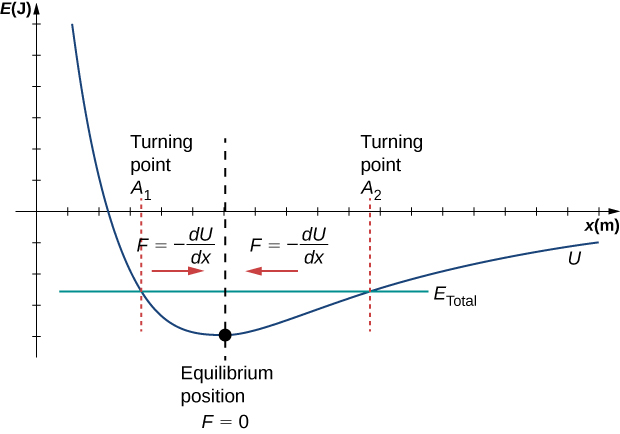Figure 15.16 The Lennard-Jones potential energy function for a system of two neutral atoms. If the energy is below some maximum energy, the system oscillates near the equilibrium position between the two turning points.

From the graph, you can see that there is a potential energy well, which has some similarities to the potential energy well of the potential energy function of the simple harmonic oscillator discussed in (Figure). The Lennard-Jones potential has a stable equilibrium point where the potential energy is minimum and the force on either side of the equilibrium point points toward equilibrium point. Note that unlike the simple harmonic oscillator, the potential well of the Lennard-Jones potential is not symmetric. This is due to the fact that the force between the atoms is not a Hooke’s law force and is not linear. The atoms can still oscillate around the equilibrium position ${x}_{\text{min}}$ because when $x<{x}_{\text{min}}$, the force is positive; when $x>{x}_{\text{min}}$, the force is negative. Notice that as x approaches zero, the slope is quite steep and negative, which means that the force is large and positive. This suggests that it takes a large force to try to push the atoms close together. As x becomes increasingly large, the slope becomes less steep and the force is smaller and negative. This suggests that if given a large enough energy, the atoms can be separated.

If you are interested in this interaction, find the force between the molecules by taking the derivative of the potential energy function. You will see immediately that the force does not resemble a Hooke’s law force $(F=\text{−}kx)$, but if you are familiar with the binomial theorem:

${(1+x)}^{n}=1+nx+\frac{n(n-1)}{2!}{x}^{2}+\frac{n(n-1)(n-2)}{3!}{x}^{3}+\cdots ,$

the force can be approximated by a Hooke’s law force.

### Velocity and Energy Conservation

Getting back to the system of a block and a spring in (Figure), once the block is released from rest, it begins to move in the negative direction toward the equilibrium position. The potential energy decreases and the magnitude of the velocity and the kinetic energy increase. At time $t=T\text{/}4$, the block reaches the equilibrium position $x=0.00\,\text{m,}$ where the force on the block and the potential energy are zero. At the equilibrium position, the block reaches a negative velocity with a magnitude equal to the maximum velocity $v=\text{−}A\omega$. The kinetic energy is maximum and equal to $K=\frac{1}{2}m{v}^{2}=\frac{1}{2}m{A}^{2}{\omega }^{2}=\frac{1}{2}k{A}^{2}.$ At this point, the force on the block is zero, but momentum carries the block, and it continues in the negative direction toward $x=\text{−}A$. As the block continues to move, the force on it acts in the positive direction and the magnitude of the velocity and kinetic energy decrease. The potential energy increases as the spring compresses. At time $t=T\text{/}2$, the block reaches $x=\text{−}A$. Here the velocity and kinetic energy are equal to zero. The force on the block is $F=+kA$ and the potential energy stored in the spring is $U=\frac{1}{2}k{A}^{2}$. During the oscillations, the total energy is constant and equal to the sum of the potential energy and the kinetic energy of the system,

${E}_{\text{Total}}=\frac{1}{2}k{x}^{2}+\frac{1}{2}m{v}^{2}=\frac{1}{2}k{A}^{2}.$

The equation for the energy associated with SHM can be solved to find the magnitude of the velocity at any position:

$|v|=\sqrt{\frac{k}{m}({A}^{2}-{x}^{2})}.$

The energy in a simple harmonic oscillator is proportional to the square of the amplitude. When considering many forms of oscillations, you will find the energy proportional to the amplitude squared.

Why would it hurt more if you snapped your hand with a ruler than with a loose spring, even if the displacement of each system is equal?

Identify one way you could decrease the maximum velocity of a simple harmonic oscillator.

### Summary

• The simplest type of oscillations are related to systems that can be described by Hooke’s law, F = −kx, where F is the restoring force, x is the displacement from equilibrium or deformation, and k is the force constant of the system.
• Elastic potential energy U stored in the deformation of a system that can be described by Hooke’s law is given by$U=\frac{1}{2}k{x}^{2}.$
• Energy in the simple harmonic oscillator is shared between elastic potential energy and kinetic energy, with the total being constant:
${E}_{\text{Total}}=\frac{1}{2}m{v}^{2}+\frac{1}{2}k{x}^{2}=\frac{1}{2}k{A}^{2}=\text{constant.}$
• The magnitude of the velocity as a function of position for the simple harmonic oscillator can be found by using
$|v|=\sqrt{\frac{k}{m}({A}^{2}-{x}^{2})}.$

### Conceptual Questions

Describe a system in which elastic potential energy is stored.

Explain in terms of energy how dissipative forces such as friction reduce the amplitude of a harmonic oscillator. Also explain how a driving mechanism can compensate. (A pendulum clock is such a system.)

The temperature of the atmosphere oscillates from a maximum near noontime and a minimum near sunrise. Would you consider the atmosphere to be in stable or unstable equilibrium?

### Problems

Fish are hung on a spring scale to determine their mass. (a) What is the force constant of the spring in such a scale if it the spring stretches 8.00 cm for a 10.0 kg load? (b) What is the mass of a fish that stretches the spring 5.50 cm? (c) How far apart are the half-kilogram marks on the scale?

It is weigh-in time for the local under-85-kg rugby team. The bathroom scale used to assess eligibility can be described by Hooke’s law and is depressed 0.75 cm by its maximum load of 120 kg. (a) What is the spring’s effective force constant? (b) A player stands on the scales and depresses it by 0.48 cm. Is he eligible to play on this under-85-kg team?

One type of BB gun uses a spring-driven plunger to blow the BB from its barrel. (a) Calculate the force constant of its plunger’s spring if you must compress it 0.150 m to drive the 0.0500-kg plunger to a top speed of 20.0 m/s. (b) What force must be exerted to compress the spring?

When an 80.0-kg man stands on a pogo stick, the spring is compressed 0.120 m. (a) What is the force constant of the spring? (b) Will the spring be compressed more when he hops down the road?

A spring has a length of 0.200 m when a 0.300-kg mass hangs from it, and a length of 0.750 m when a 1.95-kg mass hangs from it. (a) What is the force constant of the spring? (b) What is the unloaded length of the spring?

The length of nylon rope from which a mountain climber is suspended has an effective force constant of $1.40\,×\,{10}^{4}\,\text{N/m}$. (a) What is the frequency at which he bounces, given his mass plus and the mass of his equipment are 90.0 kg? (b) How much would this rope stretch to break the climber’s fall if he free-falls 2.00 m before the rope runs out of slack? (Hint: Use conservation of energy.) (c) Repeat both parts of this problem in the situation where twice this length of nylon rope is used.

### Glossary

elastic potential energy
potential energy stored as a result of deformation of an elastic object, such as the stretching of a spring
restoring force
force acting in opposition to the force caused by a deformation
stable equilibrium point
point where the net force on a system is zero, but a small displacement of the mass will cause a restoring force that points toward the equilibrium point